Effect of Shape and Parameters of Perforation in a Vertical Wellbore with Two Perforations (without Porous Media) on Pressure Drop | OMICS International
Fluid Mechanics: Open Access

# Effect of Shape and Parameters of Perforation in a Vertical Wellbore with Two Perforations (without Porous Media) on Pressure Drop

Mohammed K Salim1*, Hussein S Sultan1 and Ahmed KM AL-Shara2

1Department of Mechanical Engineering, Basrah University, Iraq

2Department of Mechanical Engineering, Misan University, Iraq

*Corresponding Author:
Salim MK
Department of Mechanical Engineering
Basrah University, Iraq
Tel:
+9647814442723
E-mail: [email protected]

Received Date: May 10, 2017; Accepted Date: June 18, 2017; Published Date: June 25, 2017

Citation: Salim MK, Sultan HS, AL-Shara AKM (2017) Effect of Shape and Parameters of Perforation in a Vertical Wellbore with Two Perforations (without Porous Media) on Pressure Drop. Fluid Mech Open Acc 4: 162. doi: 10.4172/2476-2296.1000162

Copyright: © 2017 Salim MK, et al. This is an open-access article distributed under the terms of the Creative Commons Attribution License, which permits unrestricted use, distribution, and reproduction in any medium, provided the original author and source are credited.

Visit for more related articles at Fluid Mechanics: Open Access

#### Abstract

This paper present to predict the effect of diameter and length of perforation, inflow angle of perforation, inflow velocity and shape of perforation on pressure drop and then productivity index of vertical wellbore with two perforations. In this study, a Computational Fluid Dynamics (CFD) software code ANSYS CFX15.0 has been used to simulate a model of 3-D turbulent flow with stander k−∈ in a perforated vertical wellbore. The simulation for the vertical wellbore is performed by applying the laws of conservation of mass and momentum. The effect of perforation diameter and length of perforation, inflow angle, inflow types of inlet velocity to the perforation and inflow velocity for 180º and two perforations is studied. From the results of this study, the friction pressure drop is a major component of the total pressure drop and the maximum static (friction) pressure drop occurs at the location of perforation and increases with increasing the perforation diameter. For all the perforation diameter with constant length (0.15 m), the friction pressure drop is higher than 82% of the total pressure, the acceleration pressure drop is less than 16% of the pressure drop for both numerical and theoretical results. The mixing pressure drop is about 2% of the total pressure drop for the numerical results. The increase of inflow to main flow rate ratio increasing the total pressure drop and then decreasing in productivity index.

#### Keywords

Vertical wellbore; Perforation; Pressure drop; Productivity index; Inflow angle; Inflow velocity

#### Nomenclature

A: Area (m2)

D: Wellbore Diameter (m)

d: Perforation Diameter (m)

e: Roughness (m)

l: Perforation Length (m)

Q: Main Flow Rate (m3/s)

q: Inflow Rate from Perforation (m3/s)

ui , vi: Velocity Vector

α: Angle Inflow from Perforation (deg.)

ΔP: Pressure Drop (Pa)

μ: Fluid Viscosity (kg/m.s)

ρ: Density (kg/m3)

f: Friction Factor.

#### Subscripts

1: Inlet

2: Perforation

3: Outlet

acc.: Acceleration

f: Friction

g: Gravity

i, j: Vector

m: Main Pipe

o: Unperforated

p: Perforated

T: Total

t: Total friction factor.

#### Introduction

The pressure drop occurs in many forms, such as gravity, friction, acceleration and mixing. The pressure drop is an important factor effect on the productivity index. Therefore, the total pressure drop components were analyzed severally for each term. The productivity index of vertical wellbore is defined as the amount of flow rate lifting form bottom to up corresponding the pressure energy consumed to this work. The productivity index, pressure drop and friction factor in a perforated vertical wellbore for 180º phase angle and two perforations is studied in this paper. The aim of this paper is study the parameters that cause the pressure drop to reach the best productivity index of a perforated vertical wellbore.

Number of researchers has concentrated on the effects of the perforation parameters on the production of a perforated vertical well. The effect of perforations parameters on the main flow efficiency are studied using analytical calculations. The electrolytic analog device for modeling of fluid flow through perforations also is used, which relied upon the experimental results. Later, many studies proposed numerical models based on FDM, FEM and FVM. Well productivity is the major factor which determines the economic outcome of a well, due to importance of well productivity a many researchers focused on this factor. Locke  presented a new theoretical method to predict the productivity ratio of a perforated vertical wellbore by constructing a more accurate simulation model, a finer finite element method was used to run simulations. Landman and Goldthorpe  described a mathematical model for investigating how the perforations distribution affects the performance of a perforated horizontal well producing under steady and single phase flow. The model coupled the Darcy flow into each perforation with the one dimensional momentum equation for pipe flow. Where, the overall pressure drop caused by wall friction only. A friction factor correlation to predict the effective friction factor due to wall friction and fluid inflow from perforation was proposed by Asheim et al. . They proposed a fluid flow resistance model for a perforated horizontal well theoretically. This model has been investigated experimentally, the test section pipe of (50, 100 and 150 mm) diameter contains one or two inlet perforations with 12.7 mm in diameter and 200 mm in length.

The first study effect of mixing fluid between the inflow and main flow presented by Su and Gudmundsson. They studied the effect of friction, acceleration, perforation roughness and mixing pressure drop in a perforated horizontal wellbore. Using experimental conditions on a perforated pipe, where 42.6 mm pipe diameter and 10 mm perforation diameter and the flow rate ratio larger than 0.05 with 12 SPF and 60° phase angle. Dogulu  presented a numerical model to estimate the productivity of a perforated vertical, horizontal and slanted well as a function of shot density, perforation length and phasing angle. He used FDM for a single phase and algebraic grid generation technique to build the grid of perforated well. Schulkes and Utvik  studied the total pressure drop in a perforated horizontal pipe with 56 perforations, and determined the effect of inflow on pressure drop due to acceleration and mixing through an experimental measurements. They found that the mixing pressure drop is about 10% of the friction pressure drop. Yildiz  studied a 3D analytical model to predict the productivity ratio for a perforated vertical well. The model considered various perforation phase angle, different perforation diameter and length, and formation damage around perforations.

Ansah et al.  presented a new 3D model for a vertical wellbore to predict the effects of the length of the perforation, casing (pipe) diameter, shot density and perforation phase angle, using a 3D finite element model ANSYS 5.7 to obtain a results to demonstrate the improvements of the inflow predictions. Campos et al.  developed a mathematical model to predict the effects of the friction and acceleration pressure drop on the overall productivity index of an oil well, based on the mass and momentum of conservation equations. Hagoort  presented an analytical model to predict the productivity ratio of perforated vertical wellbore, based on the analytical solution of darcy flow for a single phase in a single perforation with considered the effect of perforation damage. Fayal and Lakhdar  studied a total pressure drop due to friction and acceleration in perforated horizontal wellbore for steady and single phase flow, using CFD simulation based upon FLUENT.

Dankwa and Igbokoyi  developed an analytical method for evaluating productivity index (PI) of vertical wells in partial completion, with taken into account the effect of pressure drop, using a mathematical equation of pressure drop for a partial completion to obtain results. Abdulwahid et al.  analyzed the total pressure, static pressure and wall shear distributions along 1m of horizontal wellbore and diameter of 0.022 m, with two perforations, which the diameter for perforations is 0.006 m and length is 0.003 m, using ANSYS FLUENT and RNG k-ε model in a symmetry plane. Farajpourlar et al.  studied the major and minor pressure losses, depending on Bernoulli's principle in a single phase gas flow for vertical pipe (with and without perforation) through three images; hydrostatic, frictional and kinetic. Hua et al.  analyzed the effect of total pressure drop in a gas/liquid two phase by divided into a three components; the frictional, acceleration and mixing. Their results showed that the friction pressure drop is more than 85% of the total pressure drop, while the acceleration and mixing pressure drop are less than 15% of the total pressure drop.

#### Numerical Simulations

The greatest developments in computers hardware and software make the possibility solving theoretical simulations of difficult applications. In the present study, the theoretical simulation for 3-D turbulent flow in a perforated vertical wellbore are performed using the commercial CFD software ANSYS CFX 15.0. The simulation for the vertical wellbore is performed by applying the laws of conservation of mass and momentum with the appropriable turbulence model.

A computational procedure for the governing differential equations are explained by using finite volume method (FVM), through a solution of the continuity, momentum and turbulence model equations. The numerical analysis of the fluid flow filed is solved by using ANSYS CFX 15.0. ANSYS CFX solvers are based on the cell-vertex finite volume method. In vertex based schemes, the flow variables are stored at the vertices of the mesh elements. The fluid region is decomposed into a finite set of control volumes. Continuous partial differential equations are discretized into a system of linear algebraic equations that can be solved numerically.

#### Description of the Case Performed in the Present Study

In a current study 3-D simulations were set up and executed using ANSYS Workbench 15.0 software package. The geometry of fluid flow domain was created in Cartesian (x, y, z) coordinates using the software Design Modeler (DM) and meshed using ICEM CFD15.0 meshing software, while flow specification, solving and post-processing were all performed using CFX 15.0, with its three specialized components: CFX-Pre, CFX-Solve and CFX-Post. The geometry of the fluid flow domain consisted of a vertical pipe of 0.2 m in diameter and 1 m in length through axial centre line along the Y-axis, with two perforations perpendicular on the vertical pipe. Where diameter (d1) cutting the vertical wellbore at entrance and diminished to diameter (d2) depending on a shape of perforation through the length (lp) as shown in Figure 1.

Figure 1: Geometry of perforated vertical wellbore with two perforations and180º phase angle.

The effect of shape and parameters of perforation such as length, diameter, inflow angle and flow direction (axial and radial), and pattern (cylindrical and conical) was studied for 2 spm and 180º perforation phasing angle. The productivity index, pressure drop and friction factors of a perforated vertical wellbore can be calculated as a function of these perforating parameters.

This paper divided into three parts. The first part focus on the effect of perforation diameter and length for cylindrical perforation (axial inlet perforation) only. Five values for length and diameter of perforations which are 0.1, 0.15, 0.2, 0.25 and 0.3 m for length and 0.012, 0.018, 0.024, 0.03 and 0.036 m for diameter. While the second part is showing the effect of inflow angle on pressure drop for cylindrical perforation with 0.012 m diameter. Four values of inflow angle are studied, which are 90º, 60º, 45º and 30º. Also the effect of inflow velocity was investigated for 0.125, 0.25, 0.5 and 1 m/s axial inflow velocity (u2). The third part, for prediction of the effect of perforations shape on the static (friction) pressure drop at constant length of 0.15 m for each shape. For cylindrical shape (d1=d2=0.012 m) and conical (d1=0.012 m, d2=0.003 m), in this part the inflow velocity is radial inlet to perforation.

#### Simulation Parameters

The results of a perforated vertical wellbore with two perforation and 180º perforation phase angle simulation are presented. The simulation was performed with a consideration of water as working fluid in the wellbore and perforations. The properties of water are constant density of 1000 kg/m3, constant viscosity of 0.001 kg/m.s and constant temperature (isothermal) of 25 ºC. The total pressure drop components, friction factor and productivity index are analyzed. The grid independency and the validation for the CFD model will be viewed firstly and then the results of the simulation cases. The physical model in the present study are based on the following assumptions; (1) The direction of perforations is perpendicular on the vertical wellbore direction (Y-axis) in 3-D and (2) The fluid flow is Newtonian, turbulent, incompressible and steady state.

#### Boundary Conditions

The governing equations system in CFD can be solved only if there are boundary conditions to fulfill a solution. Hence we need to provide boundary conditions to a CFD solver. There are various forms of boundary inputs which convert a real situation to its CFD model counterpart. ANSYS CFX allows several methods for the definition of a fluid boundaries.

#### Inlet B.C

(i) From main vertical wellbore, the flow is axial.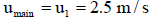(ii) From perforations, the inlet velocity is either radial or axial flow, or compound radial-axial flow to the perforation axis as shown in Figure 2.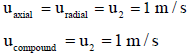#### Outlet B.C

The specified outlet relative static pressure (Ps) is zero. ANSYS CFX determines the pressure required at the inlet to maintain the specified flows.

#### Wall B.C

The velocity of the fluid at the wall boundary is set to zero (No-slip B.C), i.e. No-slip B.C eliminates all components of the velocity vector and wall is smooth.

#### Governing Equations

Fluid flow in a perforated vertical wellbore undergoes a considerable measure of physical changes such as pressure change due to friction losses in vertical pipe and perforations, mixing, acceleration and gravity, velocity change caused by varying flow regimes and density, and kinetic energy change. In order to properly description these physical changes; we need the two governing equations of fluid flow (mass and momentum equations) representation mathematical statements of the conservation of physical laws.

Conservation of mass

Based on the mass balance for the fluid element, the conservation of mass equation for an incompressible flow is given as: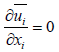(1)

Conservation of momentum

The conservation of momentum equation in Cartesian coordinates is given as: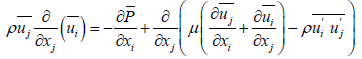(2)

Typically, called Reynolds-averaged Navier-Stokes. Where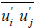: represents the Reynolds stresses or turbulent stresses tensor. To compute the Reynolds stresses we use the familiar Boussines q relationship: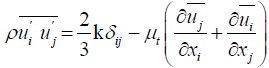(3)

Where δij represents the kronecker delta, δij=1 if i=j and δij=0 if ij.

Turbulence models (Stander k-ϵ model)

The standard k−∈ model belong to the general group of twoequation models, which tackle two separate transport equations and they are the most widely used in industrial applications because it provides economy, robustness and reasonable accuracy.

The standard k-∈ model uses the following transport equations for k;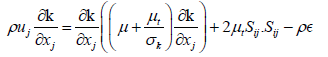(4)

And ∈;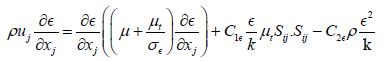(5)

Where σk and σ∈ are the Prandtl numbers connecting the diffusivities of k and ∈ to the eddy viscosity μt, the strain rate tensor can be rewritten in terms of velocity by.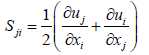(6)

It is noticeable that the transport equations include five adjustable constants;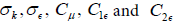. The values for these constants have been obtained by comprehensive data fitting to the standard k−ε model for a wide range of turbulent flow. These values are follows ;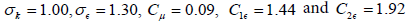#### Theoretical Model

The total pressure drop in a perforated vertical wellbore can be divided into four types. The following relationship gives these types theoretically.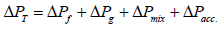(7)

In present study, the gravitational pressure drop is neglected due to remains constant along 1 m of perforated vertical wellbore. The numerical calculation includes friction and acceleration pressure drop when using the average velocity obtain from CFX directly.

Friction pressure drop

Pressure drop due to pipe wall friction caused by resistance of fluid to movement at wall pipe. The frictional pressure drop plays a major role, and it takes the largest percentage of the total pressure drop according to most researchers [4,11,17-19]. The frictional pressure in vertical wellbore can be calculated theoretically from the Darcy- Weisbach equation.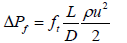(8)

This equation is used to calculate the friction pressure drop theoretically. Also friction pressure drop can be obtained from CFX directly as a static pressure difference between outlet and inlet.

Apparent friction factor (ft)

The friction factor represents the force exerted by fluid on the wall; different equations exist to calculating friction factor at wall for both smooth and roughness pipe in turbulent flow. The apparent friction factor can be written as the following equation;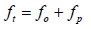(9)

The most accurate and widely accepted equation for unperforated pipe is Haaland's equation , Haaland developed an explicit universal formula for the friction factor in turbulent flow and expressed as follows;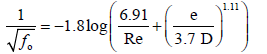(10)

The above equation is used to calculate the friction factor for unperforated wellbore. The Reynolds number represented the ratio between inertia force to viscous force. The below equation is used to calculate the Reynolds number (Re) due to average velocity of the main pipe.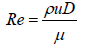(11)

Asheim et al.  presented a mathematical model to predict the local friction factor due to inflow from perforations as shown in the following equation.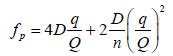(12)

Where q/Q and n are the inflow rate per unit length to main flow rate ratio and the number of perforation respectively. Asheim's et al. equation is a good acceptable to calculate the friction factor for perforation effect. The inflow rate for perforations q is expresses as;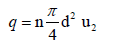(13)

Acceleration pressure drop

Pressure drop due to acceleration caused by kinetic energy changes depends on radial velocity of inflow from perforation. The acceleration pressure drop is relatively more important in small diameter than in large diameter pipe. The acceleration pressure drop can be calculated theoretically and expressed as;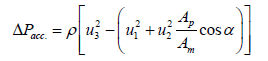(14)

The last term represented the effect of inflow angle, when the perforation direction is exactly perpendicular on vertical wellbore this term equal to zero. The reduction of inflow angle contribute to decrease the acceleration pressure drop. For numerical calculation the velocities at main inlet, inlet from perforation and outlet obtained from CFX. The outlet velocity can be calculated theoretically from conservation equation of mass for incompressible fluid, as shown below;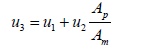(15)

The main inlet velocity and velocity inlet from perforation are specified in boundary conditions.

Mixing pressure drop

Pressure drop due to mixing caused by inflow from perforations. It arises from the interaction between inflow and main flow. When the inflow from perforation mixing with main flow, there is energy should be consumed to accelerate the inflow to the average velocity of the main pipe. The energy consumed causes pressure drop. Mixing pressure drop can be calculated from equations developed by Su and Gudmundsson  when the flow rate ratio is larger than 0.0025 and gives.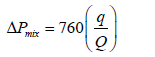(16)

It has been observed that this pressure drop reduces the production as one move from the bottom end towards the top end of the vertical wellbore.

Productivity index (PI)

Defined by the symbol PI (m3/s/pa), the mathematical expressing of productivity index is given by;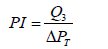(17)

#### Grid Independency Analysis

The first step of the numerical simulation is identify the maximum mesh size, which is used to solve in ANSYS CFX 15.0 comerical software. ICEM CFD 15.0 is used to generate the mesh with different maximum mesh size. Varying the maximum size of the mesh is applied to illustrate the best mesh properites which can be used to simulation the three cases in a present study. Geometry of fluid flow is a vertical pipe of 1 m in length and 0.2 m in diameter with two perforations at center vertical distance and 180º perforation phase angle, the perforation diameter is 0.012 m and length is 0.15 m.

The grid independency of all the mesh sizes are based on the average static pressure at the center line along (Y-axis), center point and fluid domain as shown in Figure 3. The minimum percentage error of the predicted average static pressure lies between the previous and the next of the maximum mesh size of 0.005 with 554168 nodes and 569148 element. The percentage error between grid 13 and grid 14 for the three locations are 0.08% for center line along (Y-axis), 0.05% for center point and 0.1%) for fluid domain. So the maximum mesh size of 0.005 is used in the simulation in order to obtained a good accuracy results. For checking quality, the determinant of element obtained equal to 0.775 at least, while the value acceptable in ICEM CFD must be greater than 0.1, then the mesh quality is good acceptable.

The flow rate at outlet computed by ANSYS CFX was compared with the exact theoretically value. For the best mesh size (maximum mesh size is 0.005), the percentage error being generally within 0.4% of the exact value. The agreement between CFD predictions and the exact solution was very good.

#### Model Validations

To validate the present study, the research of Abdulwahid et al.  is used. They used CFD to simulate a perforated pipe. A 3-D horizontal pipe with two perforations and the length of the perforation is 0.003m; diameter is 0.006 m and 180º the perforation phase angle. The center of the perforations was placed at 0.8 m downstream of the pipe from the inlet. The length of the pipe is 1 m and diameter is 0.022 m. The boundary conditions of this validation are as follow; through the axial flow (main pipe) the inlet velocity is 4 m/s and radial flow from perforation is 0.697 m/s, while the static pressure condition at the outlet equal to zero, no-slip boundary, and the wall roughness is 0.03 mm and the effect the gravity is negligible.

The ANSYS FLUENT and CFX with RNG k-ԑ model is used to simulation the fluid flow for steady state and incompressible flow. The results of this validation for static pressure drop along the center line of the pipe as shown in Figure 4 are very acceptable, and the average error between Abdulwahid et al. and present work using FLUENT is less than 2%. If using ANSYS CFX, the average error increases about 5%. The maximum value of the pressure drop appears at the entry of the pipe due to the effect of fluid entrance. A sudden pressure drop occurred at the location where the main fluid flow meets the inflow from perforations and reach the minimum value at outlet.

#### Results and Discussion

The results of this study is presented numerically by using ANSYS CFX 15.0 and theoretically by using equations which illustrated in theoretical model for 180º perforation phase angle and two perforations. The numerical and theoretical results of pressure drop as a function of perforation diameter are as shown in Figures 5 and 6 respectively. Due to higher mass inflow rate from the perforations for larger diameter which leads to more blockage to the fluid flow from the main pipe. This blockage to main flow is shown in a velocity streamline for 0.036 m perforation diameter as shown in Figure 7. The increase of inflow rate causing an increase of the pressure energy consumed to accelerate the inflow velocity up to average main velocity as shown in Figure 8, which is a contour of static pressure distribution along 1 m vertical wellbore. Thus, the total pressure drop increases with increasing the perforation diameter.

For the numerical results, the friction pressure drop is about 85 % of the total pressure drop and the acceleration pressure drop is 15%. While for the theoretical results, the friction pressure drop is about 82%, the acceleration pressure drop is 16% and the mixing pressure drop is 2% of the total pressure drop. The average error between numerical and theoretical results is about 10% for the total pressure drop.

The variation of the apparent friction factor as a function of the perforation diameter is shown in Figure 9. It's clear that the friction factor increases with increasing the perforation diameter due to increasing the mass inflow rate enter to the main pipe which causing a separation of the fluid from the wall. As a results of increasing the total pressure drop, the productivity index decrease with increasing the perforation diameter for both numerical and theoretical results as shown in Figure 10 with average error is about 10%.

The effect of perforation length of perforation on pressure drop is studied numerically for two types of inlet velocity to the perforation as illustrated in Figure 2. For axial inlet velocity to the perforation, the perforation length has no effect on the static (friction) pressure drop as shown in Figure 11. From the figure the static pressure drop remains constant for different length. Therefore, the productivity index also remains constant as shown in Figure 12.

For radial inlet velocity to the perforation, the perforation length has a significant effect on the pressure drop components due to increase surface area of inlet from perforation as shown in Figure 13. Therefore, the productivity index decreases with increasing the perforation length as shown in Figure 14. For this types of inflow from perforation, the perforation diameter is 0.012 m was considered.

The increase of mass inflow rate enters the main pipe causing increase in the generated eddies, which leads to consumed pressure energy to rotating and motion up as shown in Figure 15. This figure represents the velocity vector for radial inflow.

The effect of inflow angle on the pressure drop is studied; the inflow angle is illustrated in Figure 1. The numerical results of pressure drop for different inflow angle is shown in Figure 16. It's clear that the acceleration pressure drop increase with increasing the inflow angle and the maximum losses occurs when the perforation is horizontal (90º inflow angle). Small inflow angle contribute to decreasing the pressure drop, because the inflow rate does not constitute a heavy obstruction on flow rate coming from the main pipe. But, if the inflow angle increases the obstruction force on fluid increasing. Therefore, the productivity index decreases with increasing inflow angle as shown in Figure 17.

The main source of total pressure drop is the friction pressure drop and is about 96.5% of the total pressure and the acceleration pressure drop is 3.5%.

The total pressure drop as a function of inflow velocity for 180º perforation phase angle and two perforations and constant perforation diameter (0.012 m) is shown in Figure 18. For both numerical and theoretical results, the increase of perforation velocity will working to increasing the obstruction force for axial fluid flow from main pipe, so increasing the consuming pressure energy required to accelerate the radial inflow up to the average axial velocity of the pipe.

Also the apparent friction factor increases with increasing inflow velocity which is illustrated in Figure 19. As a result, the productivity index decreasing with increasing of inflow velocity as shown in Figure 20.

The pressure energy consumed due to inflow from perforations is illustrated in the contour of static pressure distribution as shown in Figure 21. Figure 22 shows the increase of static pressure drop along 1 m of a perforated vertical wellbore obtained from CFX for varying perforation diameter. The maximum pressure drop increase occurs at the location of the perforations due to main fluid flow obstruction by inflow from the perforations. Figure 23 shows the variation of static pressure drop along 1 m of a vertical wellbore for two shapes of the perforations. Two shapes of perforation are considered which are cylindrical and conical. Also, the variation of the static pressure drop along unperforated vertical wellbore is compared with the static pressure drop of the perforated wellbore. It's clear that the static pressure drop for unperforated vertical wellbore is less than that for the perforated wellbore for two shapes, and the static pressure drop for conical perforations is less than that for cylindrical shape due to decreasing the momentum of inflow for the conical type.

#### Conclusions

The numerical simulation of a perforated vertical wellbore have been carried out using ANSYS CFX15.0. The numerical results are compared with the theoretical results obtained from equations. This study is proposed to discuss the results of the pressure drop in a perforated vertical wellbore simulation. From the results of this study, the following conclusions were made:

(1) Increase of the perforation diameter causing an increase in the pressure drop for various perforation length.

(2) The pressure drop for conical shape of perforation is less than that for cylindrical shape at same perforation parameters.

(3) Increase of inflow velocity from the perforations causing an increase in the pressure drop.

(4) The inflow angle of the perforations has a small influence on the pressure drop.

(5) The perforation length has a negligible effect on the pressure drop for axial flow to the perforation at various perforation diameters. While, for radial flow to the perforations the pressure drop increases with increasing perforation length.

(6) For all the perforation diameter with constant length (0.15 m), the friction pressure drop is about 85% of the total pressure and the acceleration pressure drop is about 15% of the pressure drop for the numerical results. While for the theoretical results, the friction pressure drop is about 82%, the acceleration pressure drop is 16% and the mixing pressure drop is 2% of the total pressure drop.

(7) The average error between numerical and theoretical results is about 10% for the total pressure drop.

#### References

Select your language of interest to view the total content in your interested language

### Article Usage

• Total views: 2997
• [From(publication date):
June-2017 - Apr 05, 2020]
• Breakdown by view type
• HTML page views : 2796Can't read the image? click here to refresh